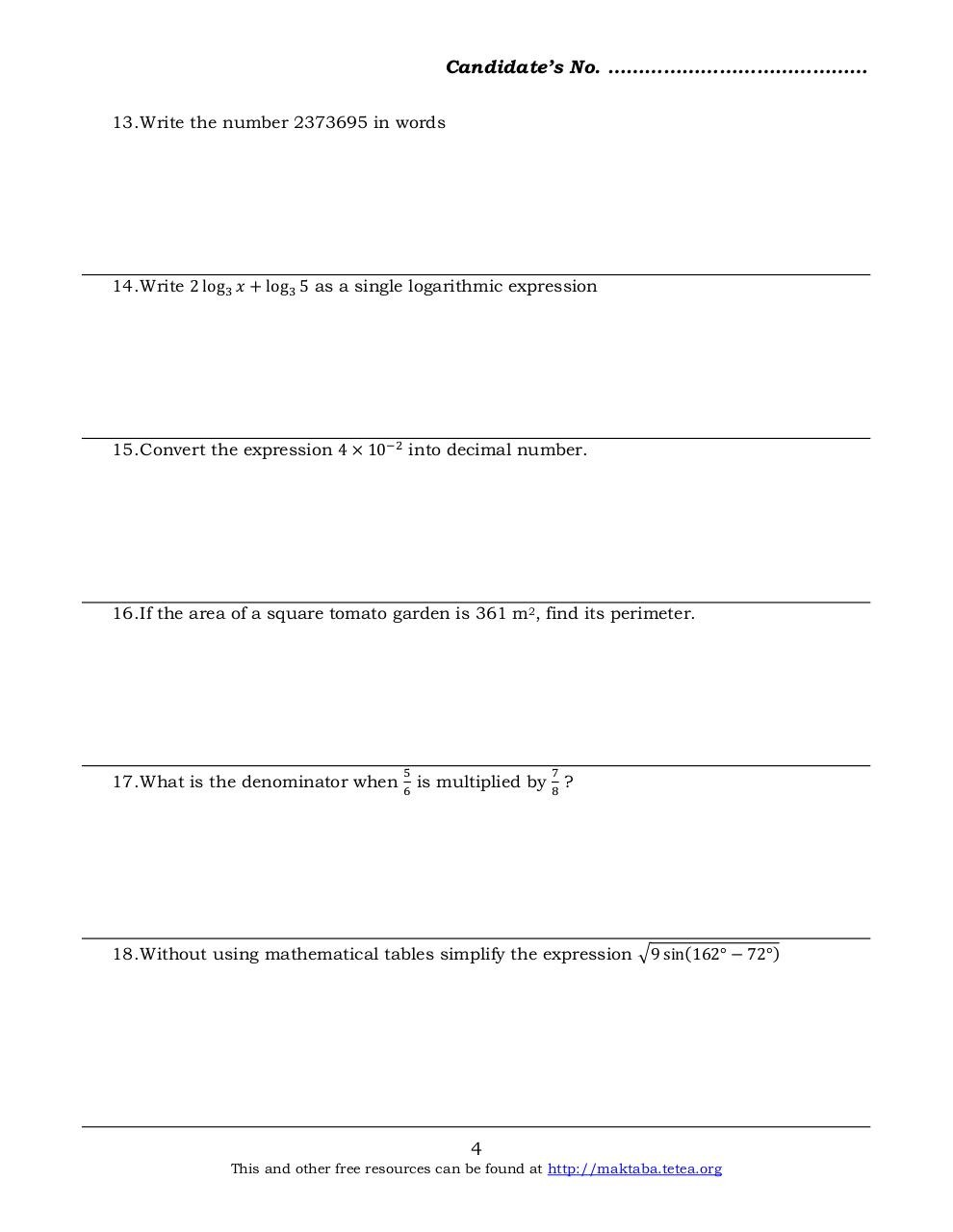# Basic Math 2013 F2.pdfPage 1 2 3 4 5 6 7 8

#### Text preview

Candidate’s No. ……………………………………
13. Write the number 2373695 in words

14. Write 2 log 3 𝑥 + log 3 5 as a single logarithmic expression

15. Convert the expression 4 × 10−2 into decimal number.

16. If the area of a square tomato garden is 361 m2, find its perimeter.

17. What is the denominator when

5
6

is multiplied by

7
8

?

18. Without using mathematical tables simplify the expression √9 sin(162° − 72°)

4

This and other free resources can be found at http://maktaba.tetea.org